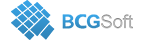BCGControlBar Pro for MFC
 void CBCGPChartSeries::SetLegendLabelContent ( BCGPChartDataLabelOptions::LabelContent content, int nDataPointIndex = `-1` )

Specifies the content to be displayed in the legend.

Call this method to customize the content that a specific data point or all data points in the series (if nDataPointIndex is -1) display in the legend.

The content can be one or combination of the following enumerated values:

• LC_DEFAULT_CONTENT = 0x0,
• LC_SERIES_NAME = 0x01,
• LC_CATEGORY_NAME = 0x02,
• LC_VALUE = 0x04,
• LC_PERCENTAGE = 0x08,
• LC_X_VALUE = 0x10,
• LC_BUBBLE_SIZE = 0x20,
• LC_Y1_VALUE = 0x40,
• LC_Y2_VALUE = 0x80,
• LC_A_TERNARY_PERCENTAGE = LC_PERCENTAGE,
• LC_B_TERNARY_PERCENTAGE = 0x100,
• LC_C_TERNARY_PERCENTAGE = 0x200,
• LC_DP_INDEX = 0x400,
• LC_A_TERNARY_VALUE = LC_VALUE,
• LC_B_TERNARY_VALUE = LC_Y1_VALUE,
• LC_C_TERNARY_VALUE = LC_Y2_VALUE,
• LC_BUBBLE_VALUES = LC_VALUE | LC_X_VALUE | LC_BUBBLE_SIZE,
• LC_ALL_BUBBLE_VALUES = LC_SERIES_NAME | LC_VALUE | LC_X_VALUE | LC_BUBBLE_SIZE,
• LC_VALUE_PERCENTAGE = LC_VALUE | LC_PERCENTAGE,
• LC_SERIES_VALUES = LC_SERIES_NAME | LC_VALUE | LC_X_VALUE,
• LC_SERIES_CATEGORY_VALUE = LC_SERIES_NAME | LC_CATEGORY_NAME | LC_VALUE,
• LC_PIE_NAME_PERCENTAGE = LC_CATEGORY_NAME | LC_PERCENTAGE,
• LC_ALL_TERNARY_VALUES = LC_A_TERNARY_VALUE | LC_B_TERNARY_VALUE | LC_C_TERNARY_VALUE,
Parameters
 content Specifies the content. nDataPointIndex Specifies a data point index, or -1 to apply the content value to the whole series.